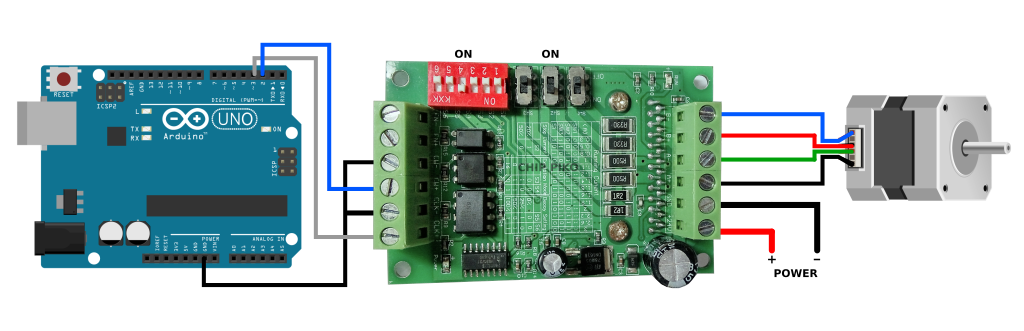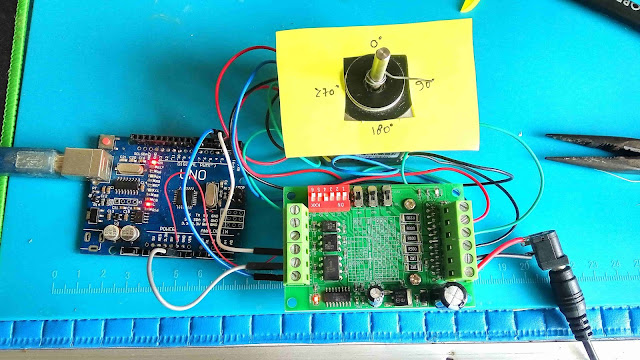How to Use the TB6560 Stepper Motor Driver

TB6560 Stepper Driver Arduino

How to Use the TB6560 Stepper Motor Driver - TB6560 is a Driver IC for stepper motors that uses PWM mode to control microsteps in bipolar motors.

The TB6560 IC is able to control the stepper, reduce vibration, rotate forward and backward and adjust the speed using only a clock signal.

TB6560 Specification:

• Power voltage 6V - 40V
• Maximum current 3A
• Excitation Modes 1/1 (2 phase), 1/2 (1-2 phase), 1/8 (2W1-2 phase) and 1/16 (4W1-2 phase)
• Capable of forward and reverse rotation
• Pin Reset and Enable
• Thermal Shutdown (TSD)
• For more details, see the datasheet below.Konfigurasi TB6460 Stepper Driver

For the stepper motor that I use in this tutorial is the Wantai Stepper Motor Nema 17 42BYGHW811. As for Power, in this article I use an adapter with a voltage of 9V and a current of 1A. You can replace it according to your needs.

Nema 17 42BYGHW811 Stepper Motor Specifications:
• Step Angle 1.8°
• Step Accuracy 5%
• 200 steps per 1 full rotation
• Working Voltage 3.1V
• Max Working Current 2.5A
• Torque 4.8 kg/cm
• BiPolar motor type
• For more details, see the datasheet below.

Arduino TB6560 Basic Wiring

To be able to control or move the stepper motor using the TB6560 driver, we must connect the Arduino to this Driver IC correctly. The circuit is as follows:Rangkaian TB6560 Arduino

Look at the picture above, I activate (On) on SW2, because I only use 0.5A current in this experiment. As for the left (red) switch, I enabled (On) only on the S4, because in this basic example, I'm going to use a 1/16 step per resolution.

If you are still confused about the step per resolution and the numbers, you can read the article Cara Kerja Motor Stepper, Jenis-jenisnya dan Mikrostepping.

Basic Code:

1. Turns Per 1°

In this program, we will try to rotate the stepper motor to be able to move from 1° to 360°, then back to 0°, and so on. Here is the short code.

int dirPin = 2;
int stepPin = 3;

//stepsPerRevolution 200 is not used in this code.
//int stepsPerRevolution = 400; //for 1/2 --> S3=1 & S4=0
//int stepsPerRevolution = 1600; //for 1/8 --> S3=1 & S4=1
int stepsPerRevolution = 3200; //for 1/16 --> S3=0 & S4=1

int sudut = 0;
int nilai = 0;
int nilaiDelay = 30;
int cekSudut = 0;
int a = 0;

void setup() {
pinMode(stepPin, OUTPUT);
pinMode(dirPin, OUTPUT);
}

void loop()
{
if (cekSudut == 0) {a = +1; }
if (cekSudut == 360) {a = -1; }

sudut = sudut + a ;
a = sudut;
nilai = map(a, 0, 360, 0, stepsPerRevolution);

//If the data is +, then rotate to the right
if (a == 1)
{
digitalWrite(dirPin, LOW);

for (int i = 0; i < nilai; i++)
{
digitalWrite(stepPin, HIGH);
delayMicroseconds(nilaiDelay);
digitalWrite(stepPin, LOW);
delayMicroseconds(nilaiDelay);
}

}

//If the data is -, then rotate to the left
if (a == -1)
{
digitalWrite(dirPin, HIGH);

for (int i = 0; i > nilai; i--)
{
digitalWrite(stepPin, HIGH);
delayMicroseconds(nilaiDelay);
digitalWrite(stepPin, LOW);
delayMicroseconds(nilaiDelay);
}
}

delay(100);
}

2. Control Using Serial Monitor

The following program scenario is to control the rotation of the stepper motor by sending the angle value through the Arduino serial monitor.

For example if we want to rotate 90°, then type 90 on the Arduino Serial Monitor and then enter. If you want to reverse 90°, type -90 then enter, and so on.Program Code Testing

//www.chippiko.com

int dirPin = 2;
int stepPin = 3;

//int stepsPerRevolution = 200; //for 1/1 --> S3=0 & S4=0
//int stepsPerRevolution = 400; //for 1/2 --> S3=1 & S4=0
//int stepsPerRevolution = 1600; //for 1/8 --> S3=1 & S4=1
int stepsPerRevolution = 3200; //for 1/16 --> S3=0 & S4=1

int dataSerial = 0;
int nilai = 0;
int nilaiDelay = 30;

void setup() {
Serial.begin(9600);
pinMode(stepPin, OUTPUT);
pinMode(dirPin, OUTPUT);
}

void loop()
{
if (Serial.available() != 0)
{
dataSerial = Serial.parseInt();
nilai = map(dataSerial, 0, 360, 0, stepsPerRevolution);

Serial.print("From Serial:");
Serial.print(dataSerial);
Serial.print(" Step:");
Serial.println(nilai);
}

//If the data is +, then rotate to the right
if (dataSerial > 0)
{
digitalWrite(dirPin, LOW);

for (int i = 0; i < nilai; i++)
{
digitalWrite(stepPin, HIGH);
delayMicroseconds(nilaiDelay);
digitalWrite(stepPin, LOW);
delayMicroseconds(nilaiDelay);
}

}

//If the data is -, then rotate to the left
if (dataSerial < 0)
{
digitalWrite(dirPin, HIGH);

for (int i = 0; i > nilai; i--)
{
digitalWrite(stepPin, HIGH);
delayMicroseconds(nilaiDelay);
digitalWrite(stepPin, LOW);
delayMicroseconds(nilaiDelay);
}
}

delay(1000);
}

If you need the TB6560 datasheet, you can view the datasheet as follows:

If you need the Nema 17 42BYGHW811 Stepper Motor datasheet, you can view the datasheet as follows: